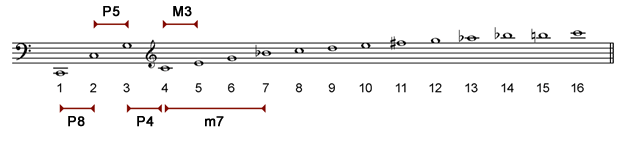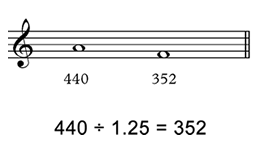When we hear a sound produced by a musical instrument we are actually listening to a multiplicity of sounds that form the harmonic series. In the seventeenth century French Joseph Sauveur (1653-1716) and the Englishman Thomas Pigot (1657-1686) noted that the strings vibrate in sections, a phenomenon that explains why one string produces this multiplicity of sounds.

The video below shows the spectrum analysis of a C two octaves below middle C (C2):

Notes:

• We have written the musical notes corresponding to the first 16 harmonics of the series. It is obvious that many harmonics follow these first harmonics.
• The first harmonic or fundamental is not necessarily the strongest harmonic.
• The balance between the harmonics varies constantly. This coupled with the immense amount of harmonics explains the difficulty we encounter in synthesizing sounds.

#### Importance of the harmonic series

The harmonic series defines many of our intervals. Listed below are the octave, fifth, fourth, major third and minor seventh:We can calculate mathematical ratio (or size) by dividing the frequencies of notes. Here we use the frequency of some harmonics to calculate the size of intervals:Interval Ratio From the harmonics
Octave 130 / 65 = 2 1 and 2
Fifth 195 / 130 = 1.5 2 and 3
Fourth 260 / 195 = 1.33 3 and 4
Major third 325 / 260 = 1.25 4 and 5
Minor seventh 455 / 260 = 1.75 4 and 7

Interestingly we can calculate the values using harmonic numbers:

Interval Ratio From the harmonics
Octave 2 / 1 = 2 1 and 2
Fifth 3 / 2 = 1.5 2 and 3
Fourth 4 / 3 = 1.33 3 and 4
Major third 5 / 4 = 1.25 4 and 5
Minor seventh 7 / 4 = 1.75 4 and 7

#### Calculating frequencies

The mathematical ratios can be used to calculate the frequency of notes. From an A 440 we calculate the frequency of C#, E and G:

 A C# (major third) E (perfect fifth) G (minor seventh) 440 440 x 1.25 = 550 440 x 1.5 = 660 440 x 1.75 = 770

If we divide by the mathematical ratio we obtain descending intervals. Here we calculate the frequency of an F, a major third below A: